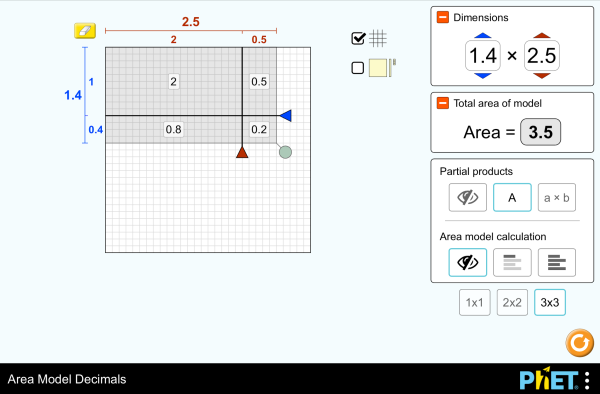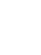# Matematički model površina - Decimalni brojeviPreuzimanje (Download)Ugradnja (Embed) zatvori Ugradi (Embed) izvršnu kopiju ove simulacije Koristi ovaj HTML za ugradnju (embed) izvršne kopije ove simulacije. Možeš mijenjati dimenzije prikaza (širinu i visinu ekrana) mijenjajući "width" i "height" atribute u HTML. Ugradi sliku. Klikom na nju pokretat će se simulacija.
Klikni i započni
Koristi ovaj HTML kod da bi se pojavio ekran sa natpisom "Klikni i započni" ("Click to Run"). Množenje Decimals Area Model PhET je podržаn od:i nаstаvnikа kаo što ste Vi!

• Množenje
• Decimals
• Area Model
• Factors
• Partial Products

### Description

Build rectangles of various sizes and relate multiplication to area. Partition a rectangle into smaller areas and discover new strategies for multiplying decimals!

### Primjeri obrazovnih ciljeva

• Recognize that area represents the product of two numbers and is additive.
• Develop and justify a strategy that uses the area model to simplify a decimal multiplication problem.
• Devise a strategy to determine the product of two rational numbers (or an integer and a rational number) by representing the product as an area or the sum of areas.
• Use an area model to understand why multiplying a given number by a decimal less than 1 results in a product smaller than the given number.
• Interpret the product of two rational numbers using base-ten tiles

### Standards Alignment

#### Common Core - Math

5.NF.B.4
Apply and extend previous understandings of multiplication to multiply a fraction or whole number by a fraction.
5.NF.B.4a
Interpret the product (a/b) × q as a parts of a partition of q into b equal parts; equivalently, as the result of a sequence of operations a × q ÷ b. For example, use a visual fraction model to show (2/3) × 4 = 8/3, and create a story context for this equation. Do the same with (2/3) × (4/5) = 8/15. (In general, (a/b) × (c/d) = ac/bd.)
5.NF.B.4b
Find the area of a rectangle with fractional side lengths by tiling it with unit squares of the appropriate unit fraction side lengths, and show that the area is the same as would be found by multiplying the side lengths. Multiply fractional side lengths to find areas of rectangles, and represent fraction products as rectangular areas.
5.NF.B.5
Interpret multiplication as scaling (resizing), by:
5.NF.B.5a
Comparing the size of a product to the size of one factor on the basis of the size of the other factor, without performing the indicated multiplication.
5.NF.B.5b
Explaining why multiplying a given number by a fraction greater than 1 results in a product greater than the given number (recognizing multiplication by whole numbers greater than 1 as a familiar case); explaining why multiplying a given number by a fraction less than 1 results in a product smaller than the given number; and relating the principle of fraction equivalence a/b = (n × a)/(n × b) to the effect of multiplying a/b by 1.
Version 1.2.1

### Teacher-Submitted Activities

Games Remote Lesson ideasTrish Loeblein HS (VŠ)
MS (SŠ)
K-5 (OŠ)
UG-Intro (VSŠ)
DZ
Remote
Matematika
Hemija
Fizika
Area Model Decimals - Grade 6Maura Dudley MS (SŠ) Guided Matematika
Middle School Math Sim AlignmentAmanda McGarry MS (SŠ) Ostalo Matematika
PRIMARIA: Alineación con programas de la SEP México (2011 y 2017)Diana López MS (SŠ)
K-5 (OŠ)
DZ
Lab
Guided
Discuss
Demo
Hemija
Astronomija
Fizika
Matematika
Jezik Downloador RunTips
Albanski All shqipModeli i Syprinës: Decimal
Arapski All العربيةنموذج مساحة الأعداد العشرية
Baskijski All EuskaraAzalera-modeloa hamartarrekin
Brazilski Portugalski All português (Brasil)Modelo de Área: Decimais
Danski All DanskArealmodel, decimaler
Engleski All EnglishArea Model Decimals
Finski All suomiPinta-alamalli desimaaleilla
Francuski All françaisModélisation par les aires avec décimales
Grčki All ΕλληνικάΥπολογισμός εμβαδού - Δεκαδικοί
Gujarati All Gujaratiઅપૂર્ણાંકમાં ક્ષેત્રફળનું મોડેલ - નિકુંજ સવાણી દ્વારા
Hindu All हिंदीनमूने का क्षेत्रफल - दाशमिक
Holandski All NederlandsOppervlakte model decimalen
Italijanski All italianoRappresentazione grafica del prodotto di numeri decimali
Japanski All 日本語面積と式の展開（小数）
Kazaški All KazakhАуданды ондық бөлшектермен есептеу
Kineski - Tradicionalni All 中文 (台灣)Area Model Decimals_面積模型與小數
Kineski - Uprošćen All 中文 (中国)面积模型：小数
Korejski All 한국어면적 모형: 소수
Letonski All LatviešuLaukuma modeļa decimāldaļskaitļi
Mađarski All magyarTerületmodell: tizedesjegyek
Mongolski All Монгол (Монгол)Талбайн Загвар Бутархайгаар
Njemački All DeutschFlächenmodell Dezimalzahlen
Persijski All فارسیمدل مساحت اعشاری ها
Poljski All polskiModel pola - ułamki dziesiętne
Ruski All русскийВычисление площади с десятичными дробями
Sinhaležanski All Sinhaleseවර්ගඑල ආකෘති දශම
Španski All españolModelo de Áreas: Decimales
Španski (Meksiko) All español (México)Modelo de Áreas: Decimales
Španski (Peru) All español (Perú)Modelo de área con decimales
Srpski All СрпскиМатематика преко површина-Децимални бројеви
Švedski All svenskaAreamodellen och decimaltal
Tamilski All Tamilமாதிரிகளின் தசமப் பரப்பளவு
Turski All TürkçeAlan Modelinde Çarpma İşlemi (Ondalık)
Ukrajinski All українськаПлощинна модель десяткових дробів
Vijetnamski All Tiếng ViệtSố thập phân với mô hình diện tích

HTML5 sims can run on iPads and Chromebooks, as well as PC, Mac, and Linux systems.

iOS 12+ Safari

Android:
Not officially supported. If you are using the HTML5 sims on Android, we recommend using the latest version of Google Chrome.

Chromebook:
The HTML5 and Flash PhET sims are supported on all Chromebooks.
Chromebook compatible sims

Windows Systems:

Macintosh Systems:

Linux Systems:

Dizajn tim Nezavisne baze i biblioteke Hvala:
• Jonathan Olson (developer)
• Karina Hensberry
• Susan Miller
• Ariel Paul
• Kathy Perkins
• almond-0.2.9.js
• base64-js-1.2.0.js
• FileSaver-b8054a2.js
• font-awesome-4.5.0
• game-up-camera-1.0.0.js
• he-1.1.1.js
• himalaya-0.2.7.js
• jama-1.0.2
• jquery-2.1.0.js
• lodash-4.17.4.js
• pegjs-0.7.0.js
• seedrandom-2.4.2.js
• text-2.0.12.js
• TextEncoderLite-3c9f6f0.js